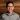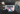Back

TechnologyOct 03, 2018

# How Functional Programming Can Help Minimize Complexity in Your Code BaseEric SuComplex functions abound in enterprise software development. We’ve all seen that function that returns different results depending on external factors, such as program state or order of method calls—or that overachieving function lined with side effects that insist on doing more than what its name suggests.

If you’ve ever had to debug such a function, it can prove to be quite difficult. Why is this? I would argue the reason is the high level of complexity involved in understanding the function. Doing so requires considering many different factors:

• When and where is the value of each local variable being changed during execution, and is the logic correct?

• What side effects does this function cause, and do these effects result in the correct application state?

• What external dependencies are influencing the result of the function, and are they a prerequisite for reproducing the error? If so, how will you go about replicating the state of these dependencies?

These are all hard questions to answer. However, there’s good news! You can avoid getting yourself into this situation altogether.

In this post, I will cover three functional programming principles that can help minimize complexity, and thus, aid in building software that is easier to reason about, test, and maintain.

#### immutability reduces complexity

One of the first concepts we are introduced to as new programmers is the good ol’ variable. We are often taught to see a variable as a container you can put a value in, and the value can be replaced with another value at any point in time.

In functional programming, variables work a little differently. Once a variable is assigned a value, that value never changes. This concept of a constant variable is very similar to that of the mathematical variable.

Consider the following equations:

##### z = x + y

Looking at these equations from a purely mathematical standpoint, it’s clear that x equals 1 and will always equal 1. We don’t expect x to change while solving for z.

We were taught to unlearn this when entering into the mystical world of programming. For example, we’ve all seen this block of code:

int x = 1 x = x + 1

Mathematically, the last statement makes no sense. x can never equal x + 1.

It turns out in the world of imperative programming, the = sign is actually not an equals sign at all, but a symbol used to “assign” a value to a variable. In functional programming, the = sign is also used to assign values to variables but is also very similar to the mathematical equals sign in that once a variable is assigned a value, it will remain that value for the life of the variable. We call this immutability.

So how does immutability help us? It reduces complexity in our code base. When following the execution path of a function, you no longer need to keep track of changing variables. In addition, immutability eliminates the possibility for race conditions, which can be an issue when running functions concurrently. This makes for safe multithreading since there is no need for resources to be protected by locks.

#### pure functions are golden

Another functional programming technique to help minimize complexity in your code base is the utilization of pure functions. For a function to be considered pure, it needs to meet the following criteria:

• The function, given the same input, must always return the same output.

• The function must not have any side effects.

You can think of a pure function as a mapping of inputs to outputs. For example, consider the following equation: y = 2x + 1. It can be said that y is a function of x, or y = f(x), where f(x) = 2x + 1. If you input 2 into f(x), you will get a result of 5—every time. Another way to look at it is that f(x) simply maps the number 2 to the number 5

##### 2 → f(x) → 5

The same concept applies to pure functions. For instance, let’s say we have a function called validateUserInput that takes in a value inputted by the user and returns true if the input is valid and false if the input is invalid. If this function is pure, a given input will always map to the same result—always. This consistency and predictability reduce the overall complexity of the function, thus making it easier to test and debug.

Side effects occur when a function modifies any state outside itself. This includes changing external variables, making network calls, accessing the database, printing to the screen, and pretty much any form of I/O. What we want to avoid is having a function unnecessarily change the state of other unrelated parts of the system upon invocation. These types of side effects lead to tight coupling and rigid code, making it harder to refactor, extend, and debug the given function.

However, not all side effects are bad—in fact, many of them are necessary. But when there are too many side effects, there is too much complexity. The same holds true that the fewer the side effects, the less complex the function.

Here is a good rule of thumb: If we can make a function pure and still get the job done, do it. What this does is eliminate the avoidable complexity and push the unavoidable complexity to the outer layers of the system, hence minimizing the footprint in which the side effects take place.

#### higher-level abstractions make manipulating data easier

Often times in functional languages, higher-level functions are provided that allow you to manipulate data in a functional and immutable way. These functions replace traditional constructs such as for-loops and while-loops, which make it very easy to mutate data and pollute the surrounding function. One very useful function implemented in most functional programming languages is map.

Consider the following:

val numbers = [1, 2, 3, 4, 5] val numbersDoubled = numbers.map(num => num * 2) print(numbersDoubled)   // [2, 4, 6, 8, 10]

As you can see, the numbers array implements a function called map, which iterates over each element in the array and passes it as an argument to the anonymous function:

num => num * 2

As the name cleverly suggests, this map function maps every element in the array (input) to the result of the provided function (output).

How would we accomplish this with a for-loop?

var numbers = [1, 2, 3, 4, 5] for(var i = 0; i < numbers.length; i++) { numbers[i] = numbers[i] * } print(numbers)    // [2, 4, 6, 8, 10]

This works, but we’re mutating data. This for-loop makes it easy for us to add more moving parts and interweave iteration logic with business logic. In short, it can get convoluted—quick. Map, on the other hand, iterates over the array, maps every element to the result of the inputted function, returns an array with the new results, and it does so without ever having to mutate data.

There are other helpful functions such as flatmap, reduce, filter, and many more, but for the sake of time, I will not get into them.

These higher-level abstractions do two things for us: 1) they ensure that we are manipulating data in a safe, immutable way, free of side effects; and 2) they abstract away all of the implementation details around iteration, allowing for more concise, readable code, and thus, making it easier to focus on the function’s true intent.

#### more functional programming

As I’ve explored the world of functional programming, I’ve discovered concepts such as immutability, pure functions, and language features like map that help me avoid unnecessary complexity within my code base. But I’ve only scratched the surface in this article. There are many other amazing aspects of functional programming that I haven’t covered, such as currying, partial application, higher-order functions, function composition, monads, functors—the list goes on. I encourage you to check out the articles below that go over these aspects of functional programming in more detail.

More on Functional Programming: